# Density theorems

(diff) ← Older revision | Latest revision (diff) | Newer revision → (diff)

The general name for theorems that give upper bounds for the number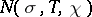of zerosof Dirichlet-functions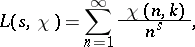where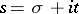and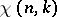is a character modulo, in the rectangle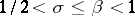,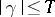. In the case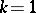, one gets density theorems for the number of zeros of the Riemann zeta-function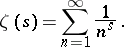The density theorems for-functions with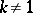are more complicated than those for the Riemann zeta-function. Asandincrease, one obtains bounds depending on these parameters. The parameterplays a decisive part in applications.

The significance of density theorems is evident from the relations enabling one to estimate the residual term in the formula for the number of prime numbersbelonging to an arithmetic progression,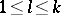,,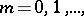and not exceeding, as a function of.

Sincedoes not increase withand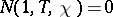, the purpose of density theorems is to obtain bounds that converge most rapidly to zero as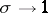. In turn, these bounds are substantially supplemented by results on the absence of zeros for Dirichlet-functions in neighbourhoods of the straight line, obtained using the Hardy–Littlewood–Vinogradov circle method. In this way it has been possible to obtain strong bounds for the amount of even numbers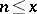that cannot be represented as the sum of two prime numbers.

Yu.V. Linnik obtained the first density theorems providing bounds forfor an individual characterand averaged bounds over all characters modulo, given. Subsequent substantial improvements of density theorems were obtained by A.I. Vinogradov and E. Bombieri, who used bounds onaveraged over all moduli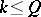and over all primitive characters modulo, given, in proving a theorem on the average distribution of prime numbers in arithmetic progressions (for). The Vinogradov–Bombieri theorem enables one to replace the generalized Riemann hypothesis in various classical problems in additive number theory. There are also various other improvements of density theorems.

How to Cite This Entry:
Density theorems. Encyclopedia of Mathematics. URL: http://encyclopediaofmath.org/index.php?title=Density_theorems&oldid=14657
This article was adapted from an original article by B.M. Bredikhin (originator), which appeared in Encyclopedia of Mathematics - ISBN 1402006098. See original article